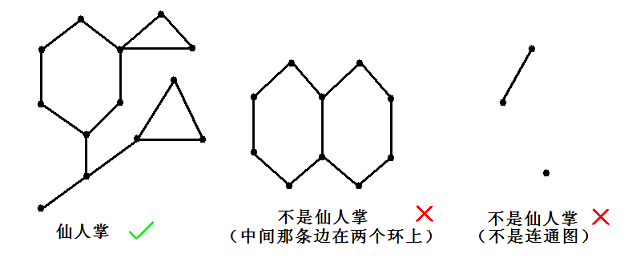#158. 【清华集训2015】静态仙人掌• 若$op=1$，表示将点$x$到根的最短路径上的所有点的状态取反（黑变白，白变黑）；
• 若$op=2$，表示将点$x$到根的最长简单路径上的所有点的状态取反；
• 若$op=3$，表示询问点$x$的子仙人掌中的黑点数目。点 $x$ 的子仙人掌定义为：删除从根到点 $x$ 的所有简单路径上的所有边后，点 $x$ 所在的连通块。

样例一

input

7 9 11
1 2
1 3
2 3
3 4
3 5
4 5
5 6
5 7
6 7
3 1
3 2
3 3
1 7
3 1
3 2
3 3
2 7
3 1
3 2
3 3



output

7
1
5
3
1
2
4
0
3



限制与约定

前七个子任务的限制

$n, q \leq 50000$。

子任务1（7分）

$n \leq 2, q \leq 2000$。

子任务2（14分）

$n \leq 20, q \leq 2000$。

子任务3（9分）

$n, q \leq 2000$。